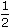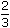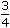# Aptitude - Ratio and Proportion - Discussion

Discussion Forum : Ratio and Proportion - General Questions (Q.No. 10)
10.
If Rs. 782 be divided into three parts, proportional to::, then the first part is:
Rs. 182
Rs. 190
Rs. 196
Rs. 204
Explanation:

Given ratio =::= 6 : 8 : 9.1st part = Rs.782 x 6= Rs. 204 23

Discussion:
92 comments Page 1 of 10.

Nabeel Ahmed said:   7 months ago
Simply;

First of all, add all three items of ratio 1/2+2/3+3/4 = 1.916,
so you need to divide 782/1.916=408.
Here 408 is on 1.
All the last multiply first item 1/2*408 = 204.
(5)

Anom said:   11 months ago
Ratios.
1/2 equal to .5
2/3 equal to .66
3/4 equal to .75.

Sum of ratio equal to 1.91
Then 1 ratio =782 /1.91 is 407.
First part ratio =0.5,
= 407 * .5 = 203.5=> i.e 204.
(2)

Aditya Rana said:   1 year ago
Well explained, thanks to all.

Anuj said:   1 year ago
How ratio is 6:8:9? Please explain.
(6)

Shravan said:   1 year ago
1/2+2/3+3/4 = 23/12.
We need first part i.e 1/2.
1/2 * 12/23 * 782 = 204.
(5)

Prajajwl said:   2 years ago
LCM OF 2,3,4 IS 12 THEN;

1/2 x 12 = 6 that's how it is done same to all u get.
6,8,9 then the sum of 8, 9, 6 is 23 that's how the answer comes first part 6/23 x 782 = 408.
(5)

Poojitha said:   2 years ago
1/2 + 2/3 + 3/4 = 23/12 we are doing this because they gave a + b + c = 789.

So, we have to calculate a ,b , c values individually and at last we want a value so (a/total * given) == 1/2 (because we want 1st part value) Ã· 23/12 after solving this we get 6/23 now 6/23 * 789(given).

Nikita Salunkhe said:   2 years ago
Solution:

1/2 = 0.5, 2/3 = 0.67 , 3/4 = 0.75.
After this Let's consider common factor k, so this becomes,
0.5k, 0.67k , 0.75k.

Add this 0.5k + 0.67k + 0.75k = 782.
Find k -
k=407.29.

And we want to derive 1st no so put the value of k into 0.5k, which becomes
0.5 * 407.29= 203.64 ~ 204.

Samir said:   2 years ago
1/2:2/3:3/4.
6,8,9.
1st part , 782 * 6 / 23 = 204 ( simple answer).

Simron said:   2 years ago
According to me, the solution is;

First, calculate the ratio= 1/2+2/3+3/4 = 23/12.
Now, we know the total is 782 Rs.
So the 1st part will be 783*1/2*12/23 = 204 Rs.
(1)